A semi-curated blog of computer graphics and rendering.

This is a blog post from the past. Originally written at 2020-02-12.

Mandelbrot! The “Hello world” of GFX programming. You may also have noticed the Mandelbrot Set image from my blog’s intro. Beautiful, mesmerizing, infinite. Buti such is the beauty of math. And as the tutorials are a little bit scarce, this actually took more time than I thought, and it is also easier than I thought! Featured image by myself (yay!).

## Introduction

As we all know, Mandelbrot Set belongs to a category named “fractal”; and as Grant from 3Blue1Brown explained, Fractals are typically not self similar. A fractal is just a shape with non-integer dimension, and it won’t be smooth, even if you zoom in forever.

## Julia Set

Now before everything, we should learn about Julia set. Why? You will know a little bit later.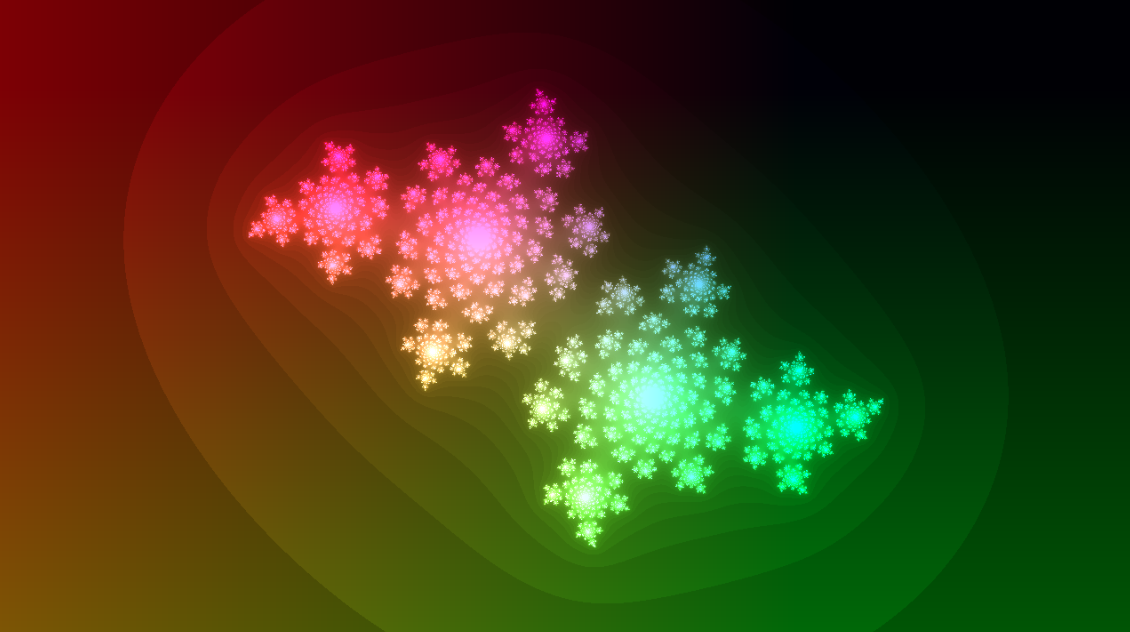Now, this is not so worse than the Mandelbrot set, right? Bear with me, and we will first start with a teeny tiny concept. First, we get an empty canvas: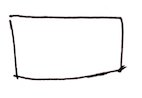Then, for every pixel on this canvas, we normalize its position to (-1, 1), then transfer its position to the complex plane. After the transformation, (0.3, 0.3) should be 0.3 + 0.3i, and (0.1, -0.5) should be 0.1 - 0.5i. That isn’t hard at all, right? Now that every pixel’s position was transformed to its complex plane number, we will name this complex plane variable z, and perform the following, easy peasy maths to it:

$z = z^2$

lots of times. What does that mean? Well, let’s pick a pixel position at random, say (0.1, 0.1). After the complex plane transformation, the pixel position should now be 0.1 + 0.1i. And after this simple computation, the new z should be

\begin{align} 0.1^2 + 0.1i^2 + 2\times 0.1 \times 0.1i\\ = 0.01 - 0.01 + 0.02i\\ = 0.02i \end{align}

Well, that’s good and all, but let’s perform another $$z = z ^ 2$$ on it, OK? And another $$z = z ^ 2$$? And another $$z = z ^ 2$$? And another $$z = z ^ 2$$? ……

After enough $$z = z ^ 2$$, we will find out numbers will have two different results:

1. Sprialing to infinity
2. Loop forever, or spiraling to zero… (aka not spiraling to infinity)

Now let’s color the points which spirals to infinity to black, & others to white. And as this is a per-pixel iteration process which requires a lot of computation, of course we are gonna use GLSL. As GLSL doesn’t really support complex number computation, we can fake it with

$p = (p_x^2 - p_y^2, 2p_x p_y)$

Think about it. As we treat the y coordinate as complex number, that means $$y^2 = -1$$, right? OK, GLSL time!

float julia(vec2 uv) {
int i;
for (i = 0; i < 100; i++) {
uv = vec2(uv.x * uv.x - uv.y * uv.y,
2.0 * uv.x * uv.y);
if (length(uv) > 100.0) {
break; // Spiraling to infinity; stop
}
}
return float(i) / 100.0;
}


Let’s take a look at this function before appreciating the result. We can guess that in this huge amount of loop, the uv might be bouncing all around (condition 1); and when uv is getting too far, we can treat it as approaching infinity already, and thus cutting the loop off. In other words, when the $$z$$ transformation was stuck in loop forever, then even after 100 loops the uv won’t get very big (condition 2).

Thus if i is 100, that means it might be stuck in a loop; or it takes so much calculation it doesn’t really get to infinity yet. In either case, we are just gonna pretend that it is, indeed, stuck. And if i is less than 100, that means after all these transformations, uv was shot to infinity. And the result?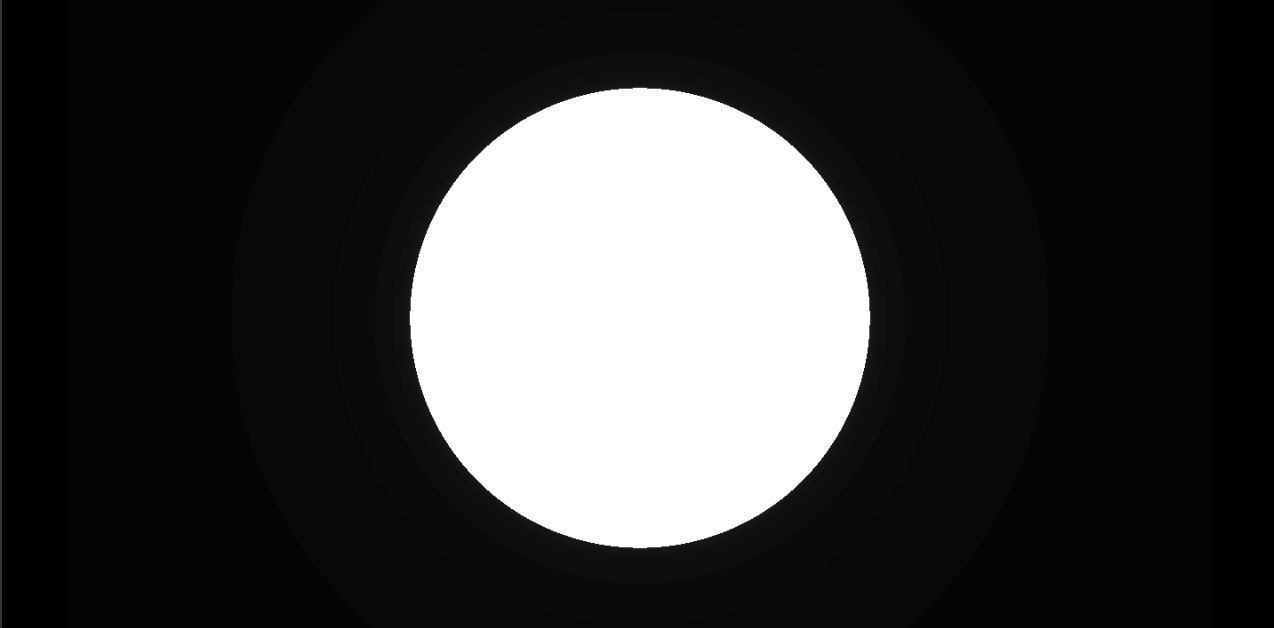Just a boring circle. This does not look like those awesome julia sets at all! Well, just wait for a moment and let’s change this

$z = z^2$

to

$z = z^2 + c$

in which c is a random number. For the sake of fun, how about 0.25?

float julia(vec2 uv) {
int i;
float c = 0.25;
for (i = 0; i < 100; i++) {
uv = vec2(uv.x * uv.x - uv.y * uv.y + c,
2.0 * uv.x * uv.y);
if (length(uv) > 100.0) {
break; // Spiraling to infinity; stop
}
}
return float(i) / 100.0;
}


And here’s what we get:This looks like a four-leave clover. It is weird, right? A circle could be so seriously deformed by just adding a c after the x component. It is not just that, also; this thing could be zoomed in forever. Let’s zoom!As we can see, the patterns are self-similar here: after zooming in, we get more of these little things, and it could go on forever. That’s what make fractals cool. It’s like looking at a microscopic microscopic microscopic world. It also makes you think. What about adding a constant to y component? Say, 0.5?

float julia(vec2 uv) {
int i;
vec2 c = vec2(0.25, 0.5);
for (i = 0; i < 100; i++) {
uv = vec2(uv.x * uv.x - uv.y * uv.y + c.x,
2.0 * uv.x * uv.y + c.y);
if (length(uv) > 100.0) {
break; // Spiraling to infinity; stop
}
}
return float(i) / 100.0;
}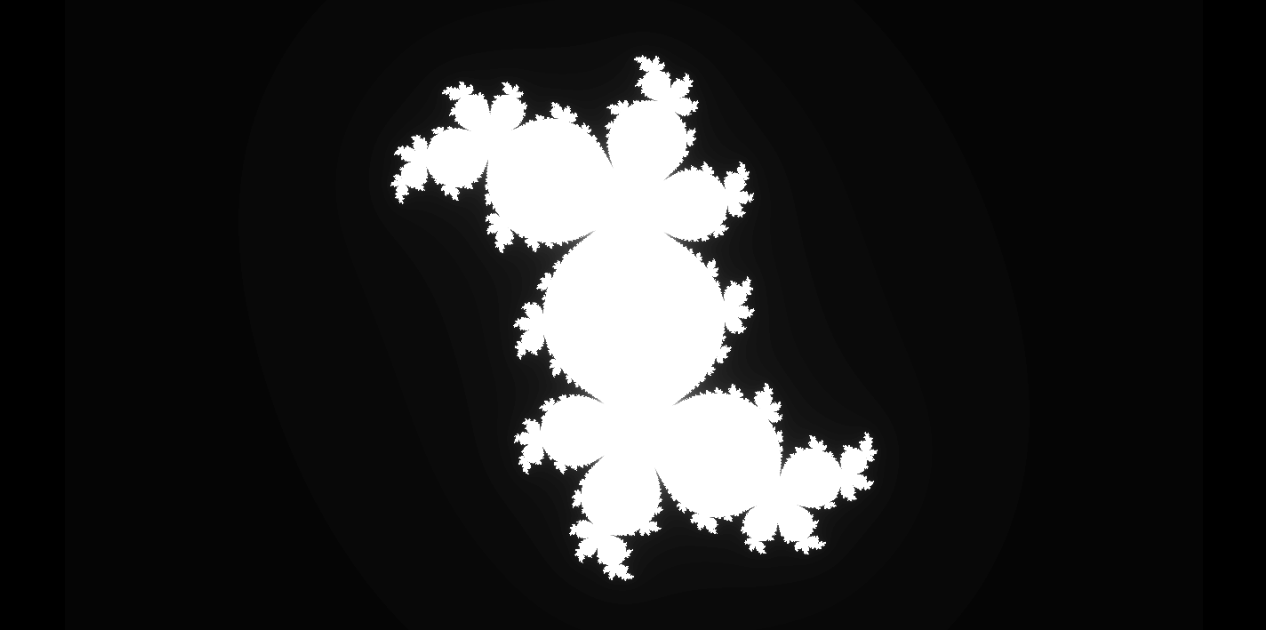Woah there! Now this is a really cool shape! And this, my friend, is the Julia set! It is just

$z = (z + c)^2$

Calculated over and over again for every single pixel. The featured image and others are just a little special effects I added on my own; go figure it out on your own! It’s fun! By setting the c bigger, the whole set will begin to disintegrate. But things are at their most beautiful before their total destruction: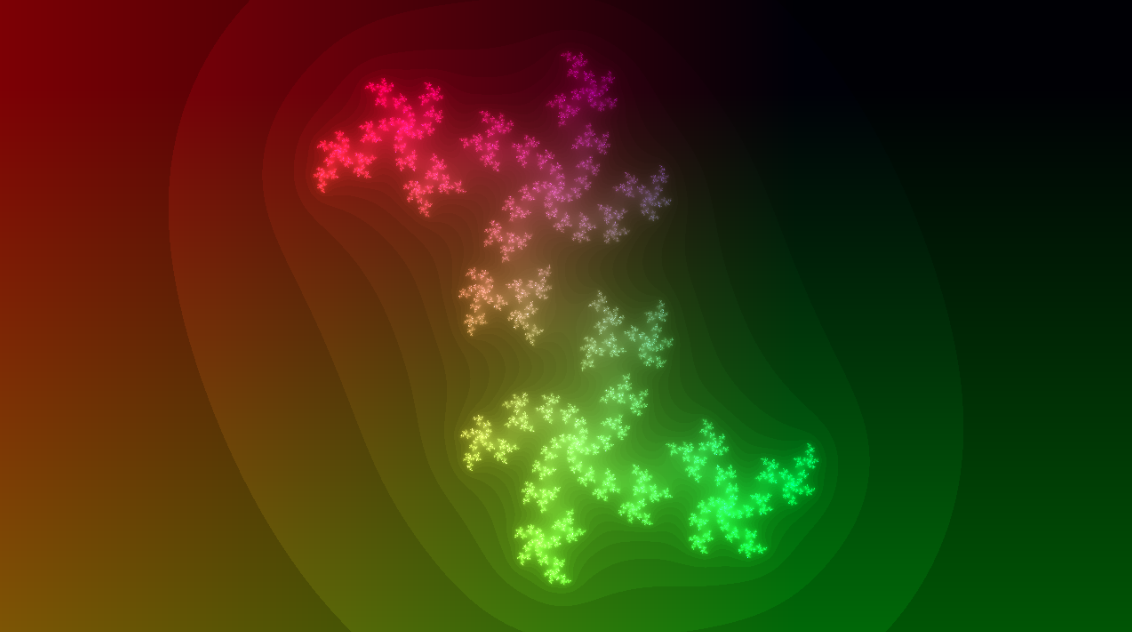(c = (0.25, 0.6))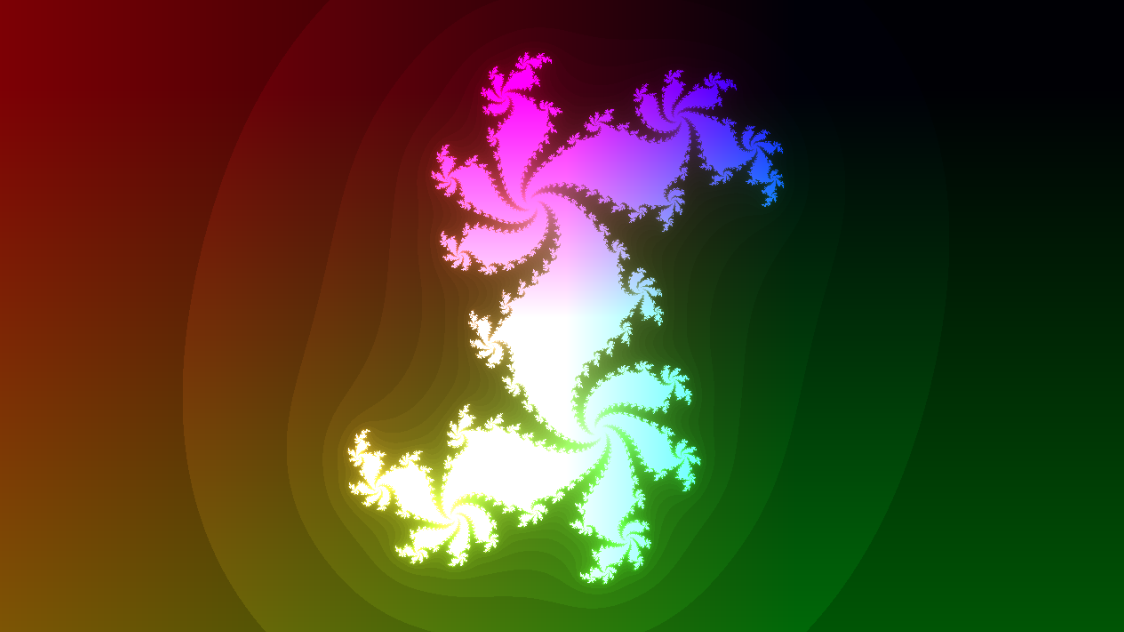(c = (0.37, -0.35))

## Mandelbrot set

Mandelbrot is this guy who coined term “fractal”. And one day he loved Julia set so much, he wanted to know every possible valid Julia set. So he updated the julia function:

float julia(vec2 uv, vec2 c) {
int i;
for (i = 0; i < 100; i++) {
uv = vec2(uv.x * uv.x - uv.y * uv.y + c.x,
2.0 * uv.x * uv.y + c.y);
if (length(uv) > 100.0) {
break;
}
}
return float(i) / 100.0;
}


So now Mandelbrot could invoke the Julia function by using julia(uv, vec2(0.1, 0.1)) to check out the shape, which is really convinient.

Then he thought screw it, why not just insert uv as c, and the original uv parameter to be vec2(0.0, 0.0)? In this way, we could know whether (0, 0) spirals out at all possible Julia combinations. And so he did it:

float f = julia(vec2(0.0, 0.0), uv);


And BAM! Mandelbrot Set!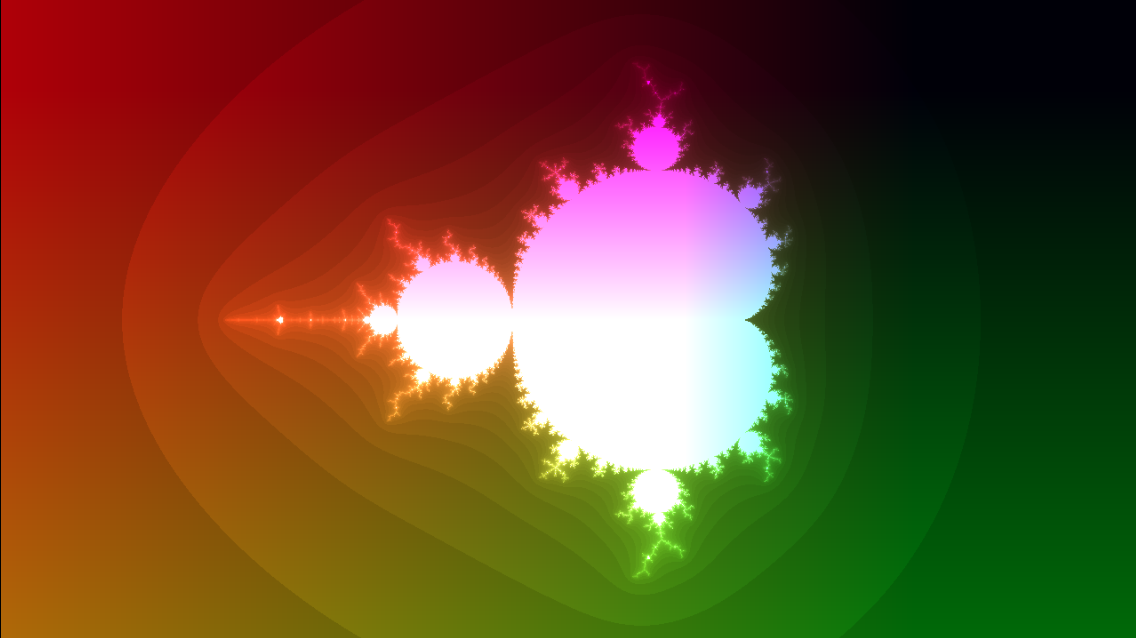White areas means the (0, 0) does not spiral out when $$c = uv$$. So all positions within the white area are valid Julia sets! Yay! (And a little bit of positions outside the white area too, because not all Julia set’s (0, 0) are white).

Mandelbrot set is beautiful, it could be zoomed in forever and does not have a single problem (theoretically; your GPU might vomit at all those floating points). It’s like a kaleidoscope of infinity; deep down, you will find out that it is, indeed, self similar. There are a Mandelbrot set deep within itself. You just need to zoom in!

## Conclusion

We saw Mandelbrot today, the beauty of maths. It’s a graph plotted by numbers that doesn’t even exist in real life. Of course there are other mathy stuffs which is the ugliness of maths, but I guess we are not gonna cover it today (*cough* calculus *cough*).